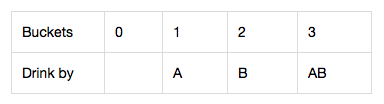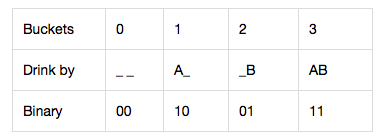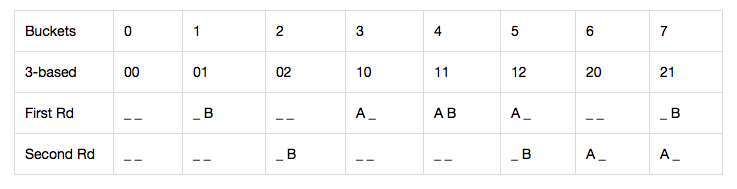Related Articles
Minimum number of pigs required to find the poisonous bucket
• Difficulty Level : Medium
• Last Updated : 20 Apr, 2021

Given an integer N denoting the number of buckets, and an integer M, denoting the minimum time in minutes required by a pig to die after drinking poison, the task is to find the minimum number of pigs required to figure out which bucket is poisonous within P minutes, if there is exactly one bucket with poison, while the rest is filled with water.

Examples:

Input: N = 1000, M = 15, P = 60
Output: 5
Explanation: Minimum number of pigs required to find the poisonous bucket is 5.

Input: N = 4, M = 15, P = 15
Output: 2
Explanation: Minimum number of pigs required to find the poisonous bucket is 2.

Approach: The given problem can be solved using the given observations:

• A pig can be allowed to drink simultaneously on as many buckets as one would like, and the feeding takes no time.
• After a pig has instantly finished drinking buckets, there has to be a cool downtime of M minutes. During this time, only observation is allowed and no feedings at all.
• Any given bucket can be sampled an infinite number of times (by an unlimited number of pigs).

Now, P minutes to test and M minutes to die simply tells how many rounds the pigs can be used, i.e., how many times a pig can eat. Therefore, declare a variable called r = P(Minutes To Test) / M(Minutes To Die).

Consider the cases to understand the approach:

Case 1: If r = 1, i.e., the number of rounds is 1.
Example: 4 buckets, 15 minutes to die, and 15 minutes to test. The answer is 2. Suppose A and B represent 2 pigs, then the cases are:Obviously, using the binary form to represent the solution as:Conclusion: If there are x pigs, they can represent (encode) 2x buckets.

Case 2: If r > 1, i.e. the number of rounds is more than 1. Let below be the following notations:

• 0 means the pig does not drink and die.
• 1 means the pig drinks in the first (and only) round.

Generalizing the above results(t means the pig drinks in the t round and die): If there are t attempts, a (t + 1)-based number is used to represent (encode) the buckets. (That’s also why the first conclusion uses the 2-based number)

Example: 8 buckets, 15 buckets to die, and 40 buckets to test. Now, there are 2 (= (40/15).floor) attempts, as a result, 3-based number is used to encode the buckets. The minimum number of pigs required are 2 (= Math.log(8, 3).ceil).Below is the implementation of the above approach:

## C++

 `// C++ program for the above approach` `#include ``using` `namespace` `std;` `// Function to find the minimum number of pigs``// required to find the poisonous bucket``void` `poorPigs(``int` `buckets,``              ``int` `minutesToDie,``              ``int` `minutesToTest)``{``    ``// Print the result``    ``cout << ``ceil``(``log``(buckets)``                 ``/ ``log``((minutesToTest``                        ``/ minutesToDie)``                       ``+ 1));``}` `// Driver Code``int` `main()``{``    ``int` `N = 1000, M = 15, P = 60;``    ``poorPigs(N, M, P);` `    ``return` `0;``}`

## Java

 `// Java program for the above approach``import` `java.io.*;` `class` `GFG``{` `  ``// Function to find the minimum number of pigs``  ``// required to find the poisonous bucket``  ``static` `void` `poorPigs(``int` `buckets, ``int` `minutesToDie,``                       ``int` `minutesToTest)``  ``{` `    ``// Print the result``    ``System.out.print((``int``)Math.ceil(``      ``Math.log(buckets)``      ``/ Math.log((minutesToTest / minutesToDie)``                 ``+ ``1``)));``  ``}` `  ``// Driver Code``  ``public` `static` `void` `main(String[] args)``  ``{``    ``int` `N = ``1000``, M = ``15``, P = ``60``;``    ``poorPigs(N, M, P);``  ``}``}` `// This code is contributed by Dharanendra L V.`

## Python3

 `# Python program for the above approach``import`  `math` `# Function to find the minimum number of pigs``# required to find the poisonous bucket``def` `poorPigs(buckets, minutesToDie, minutesToTest):``  ` `    ``# Prthe result``    ``print``(math.ceil(math.log(buckets)\``                    ``/``/` `math.log((minutesToTest \``                                 ``/``/` `minutesToDie) ``+` `1``)));` `# Driver Code``if` `__name__ ``=``=` `'__main__'``:``    ``N ``=` `1000``;``    ``M ``=` `15``;``    ``P ``=` `60``;``    ``poorPigs(N, M, P);` `# This code is contributed by 29AjayKumar`

## C#

 `// C# program for the above approach``using` `System;``class` `GFG``{` `  ``// Function to find the minimum number of pigs``  ``// required to find the poisonous bucket``  ``static` `void` `poorPigs(``int` `buckets, ``int` `minutesToDie,``                       ``int` `minutesToTest)``  ``{` `    ``// Print the result``    ``Console.WriteLine((``int``)Math.Ceiling(``      ``Math.Log(buckets)``      ``/ Math.Log((minutesToTest / minutesToDie)``                 ``+ 1)));``  ``}` `  ``// Driver Code``  ``static` `public` `void` `Main()``  ``{``    ``int` `N = 1000, M = 15, P = 60;``    ``poorPigs(N, M, P);``  ``}``}` `// This code is contributed by jana_sayantan.`

## Javascript

 ``
Output:
`5`

Time Complexity: O(1)
Auxiliary Space: O(1)

Attention reader! Don’t stop learning now. Get hold of all the important mathematical concepts for competitive programming with the Essential Maths for CP Course at a student-friendly price. To complete your preparation from learning a language to DS Algo and many more,  please refer Complete Interview Preparation Course.

My Personal Notes arrow_drop_up